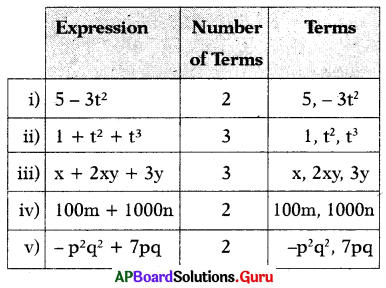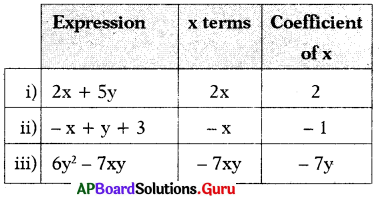# AP Board 7th Class Maths Solutions Chapter 9 Algebraic Expressions Ex 9.1

SCERT AP 7th Class Maths Solutions Pdf Chapter 9 Algebraic Expressions Ex 9.1 Textbook Exercise Questions and Answers.

## AP State Syllabus 7th Class Maths Solutions 9th Lesson Algebraic Expressions Ex 9.1

Question 1.
Write numerical and algebraic coefficients of the following terms.
(i) 4xy
Given the term is 4xy
Numerical coefficient: 4
Algebraic coefficient: xy(ii) – 7a2 b3 c
Given term is – 7a2 b3 c
Numerical coefficient: – 7
Algebraic coefficient: a2b3c

(iii) $$\frac{p q}{2 r}$$
Given terra is $$\frac{p q}{2 r}$$
Numerical coefficient: $$\frac{1}{2}$$
Algebraic coefficient: $$\frac{p q}{r}$$

(iv) – 6mn
Given term is – 6mn
Numerical coefficient: – 6
Algebraic coefficient: mn

Question 2.
Write the number of terms in each of the following expressions and write ’ them:
(i) 5 – 3t2
(ii) 1 + t2 + t3
(iii) x + 2xy + 3y
(iv) 100m + 1000n
(v) – p2q2 + 7pqQuestion 3.
In – 5ab2c write the coefficients of
(i) b2c
(ii) – b2c
(iii) – 5abc
(iv) 5ac
(v) ab2
(vi) – 5ab
Given term is – 5ab2c
(i) Coefficient of b2c is – 5a
(ii) Coefficient of – b2c is 5a
(iii) Coefficient of – 5abc is b
(iv) Coefficient of 5ac is – b2
(v) Coefficient of ab2 is – 5c
(vi) Coefficient of – 5ab is bc

Question 4.
Write term containing x and coefficient of x for the following algebraic expressions.
(i) 2x + 5y
(ii) -x + y + 3
(iii) 6y2 – 7xy